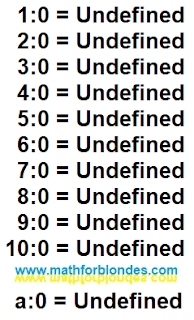## 8/17/2010

### Table division by zero

Division by zero is forbidden. Any number, positive or negative, whole or shot, to divide by zero is forbidden. Mathematicians say that the result of division by zero is undefined. Therefore a division table by zero will look so:Table division by zero

If to designate any number through а, then a division table by zero for any numbers will consist only of one line (last line).

Why is the result of division by zero undefined? Because any number multiplied by zero equals zero. Since the mathematical operation of division is the inverse of the mathematical operation of multiplication, division by zero results in all the numbers at once. And mathematicians want to have one specific number.

Is it possible to solve the problem of division by zero? Yes, this problem can be solved. As a result of dividing by zero of a specific number, you will get another specific number. But this requires a much better understanding of mathematics.

I solved this problem. But at the same time, my views on some of the statements of mathematicians have changed dramatically. I spent a lot of time on this. Here you can compare your views on mathematics with the way I see it.